Next: 7.1.1 Wireless Applications Up: 7. Simulation Studies Previous: 7. Simulation Studies

# 7.1 Technology A: Pseudomorphic HEMT on GaAs

In Fig. 7.2 the output characteristics of a pseudomorphic Al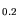Ga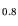As/ InGaAs/GaAs HEMT is shown in comparison with measurements. Generation/recombination and self-heating are included in these simulations. These two effects allow to match the output conductance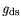correctly for a wider bias range than previously reported in . Self-heating reduces the drain current and the transconductance. The generation/recombination mechanisms cause an effective modification of the gate potential to more positive values. This modification depends on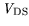, so that the output conductance is modified even for lowvoltage. More details are given with the power HEMT in the next section.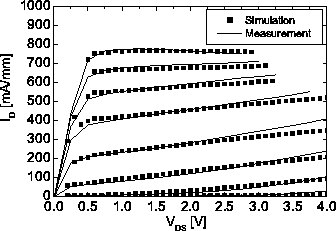In Fig. 7.3 the simulated gate length scaling is shown in comparison with measurements for different devices investigated in this technology.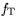is calculated based on (4.11) and the equivalent circuit from Fig. 4.1. Within the process variations a product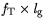= 14.5-15.5 GHz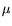m is observed at= 1.5 V. A logarithmic scale is applied and an almost linear increase can be seen. Variations occurring are due to a slightly changing aspect ratio, i.e. a changing ratio of gate length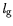and gate-to-channel separation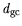.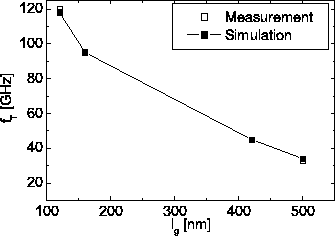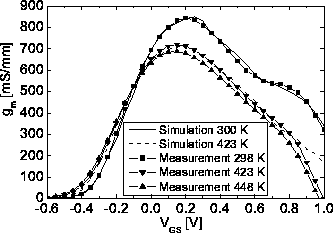In Fig. 7.4 the measured dependence of the transconductance on temperature between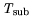= 300 K and= 473 K is shown. The decrease of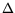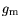/for this on-wafer situation amounts to 100 mS/mm/100 K. The overall reduction for the observed temperature range amounts to 21.5%. The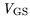voltage for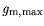changes to more negative values. This effect can be correlated to a balance of the effective reduction of the Schottky barrier height and bias shift due to the increase of the parasitic resistances. The simulations are included for= 300 K and= 423 K. The temperature dependent simulation can precisely reproduce thecurve for both temperatures except for the decrease at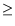1 V, where the simulation starts to overestimate thefor both temperatures. This results from the fact that self-heating is only modeled with fitted boundary conditions, as explained in Chapter 6. To match thecurve to such high precision the upper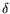-doping was adjusted to fit the drain current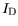, the transconductance, and the threshold voltagein agreement with the statistical analysis of Chapter 5.

For= 300 K a second peak of the transconductance is visible, which is due to the parasitic MESFET, i.e., additional current flow in the AlGaAs barrier layer. For higher temperatures the second peak reduces. The occurrence of a pronounced second peak, i.e., an increase ofwithforforis due to a number of conditions. In principal thecan be understood to be composed of twocurves. The first is the regular HEMT channel curvewith current flow exclusively in the channel. This produces thepeak and has a sharp drop forfor. As was seen in Chapter 3, Real Space Transfer (RST) is also responsible for the reduction of this first peak relative to an ideal situation, where no real space transfer at all is possible.

The second peak is composed of contributions from current flow in the barrier material AlGaAs, which results in a secondcurve with afor. The distance between these two peaks is now related to various physical quantities, but most important to:

• the Schottky barrier height.
• the relation of channel layer mobility to barrier layer mobility (see Fig. 3.1).
• the band edge discontinuity channel/barrier.
• the ohmic contact situation.

In the investigated device in Fig. 7.4 the ohmic contact situation is similar to Case II in Fig. 3.25, i.e., the channel is not directly contacted. Thus,is reached for some bias which is determined by the doping and the gate-to-channel separation. The current transfer into the spacer and barrier is dominated by RST. In comparison with a directly contacted channel (Case I in Fig. 3.25),foris relatively more positive due to the additional line resistance of the caps. At the same time, the utmost right position for the second peak is limited by the opening gate-diode at=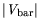(barrier height), in this case about= 0.7 V. Due to the relatively positive, the parasitic MESFET behavior, i.e., current flow in the barrier, occurs atbias close to the, where the gate-diode opens, which is not necessarily the case for a HEMT with a more negative threshold voltage. To the right the peak is limited by the opening diode, since the opening diode leads to a drastic reduction of the current gain. As a consequence, a peak position is visible for this particular case.

This argumentation can also explain the temperature dependence. Effectively three temperature effects occur for rising temperatures: the effective Schottky barrier height decreases, the band edge discontinuity decreases, and the overall effective carrier velocity drops in both, the channel and the barrier.

The effective barrier height reduction shifts the peak of the parasitic MESFET to more negative values, while the sum of the twocontributions drops. Thus, thecurve looks more homogeneous, although it still consists of the two contributions.

SubsectionsNext: 7.1.1 Wireless Applications Up: 7. Simulation Studies Previous: 7. Simulation Studies
Quay
2001-12-21